This article is a companion article to my another post Generalized Linear Model. In this article, I will implement some of the learning algorithms in Generalized Linear Model. To be more specific, I will do some examples on linear regression and logistic regression. With some effort, google search gives me some very good example data sets to work with. The datasets collected by Larry Winner is one of the excellent sets, which will be used in the article.

The implementations here use Python. Required 3rd party libraries are:

## Linear Regression

The first example is to predict monthly production cost according to production output for a hosiery
mill. The data set is here, with description here. The first 3 rows of the data look like:

  1   46.75   92.64
2   42.18   88.81
3   41.86   86.44


The first column represents month, the second column represents production output and the last column represents production cost.

import requests
import re
import matplotlib.pyplot as plt
import numpy as np

# obtain sample data
millcost_url = 'http://www.stat.ufl.edu/~winner/data/millcost.dat'
response = requests.get(millcost_url)
data_pat = '(\d+\.\d+)' # regular expression to extract data
data = map(float, re.findall(data_pat, response.content))
x, y = data[::2], data[1::2]
m = len(x)
print('Number of sample points: {}'.format(m))
print('The first three sample points:')
print(zip(x, y)[:3])

Number of sample points: 48
The first three sample points:
[(46.75, 92.64), (42.18, 88.81), (41.86, 86.44)]


We have 48 sample points, let's try to plot these sample points for visualization.

plt.plot(x, y, 'ro')
plt.show()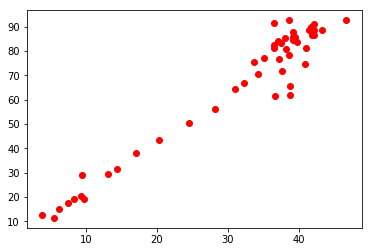Apparently, linear regression will be our choice. In this example, we have only one feature: production output, which will be represented by variable $x$. Then the learning algorithm for the production cost $y$ is

$$y = \theta_1 x + \theta_0.$$

If we introduce a dummy feature $x_0=1$, then the learning algorithm will be in the standard form

$$y= h_\theta = \left[\begin{array}{c}\theta_1 \\ \theta_0 \end{array}\right]^T \left[\begin{array}{c}x \\ 1 \end{array}\right],$$

where

$$\theta = \left[\begin{array}{c}\theta_1 \\ \theta_0 \end{array}\right]$$

is the learning parameter. In order to determine $\theta$, we need to minimize

$$\begin{split} J(\theta) &= \frac{1}{2}\sum_{i=1}^{m}\left(y^{(i)}-h_\theta(x^{(i)})\right)^2 \\ &= \frac{1}{2}\sum_{i=1}^{m}\left(y^{(i)}-\theta_1 x^{(i)} - \theta_0\right)^2. \end{split}$$

Here $m$ is the size of the sample set. The gradient $J$ is

$$\begin{split} \frac{\partial J}{\partial \theta_1} &= \sum_{i = 1}^m \left(y^{(i)}-\theta_1 x^{(i)} - \theta_0\right)(-x^{(i)}) \\ &= \left(\sum_{i=1}^m \left(x^{(i)}\right)^2\right) \theta_1 + \left(\sum_{i=1}^m x^{(i)}\right) \theta_0 - \left(\sum_{i=1}^m x^{(i)}y^{(i)}\right) \\ \\ \frac{\partial J}{\partial \theta_0} &= \sum_{i = 1}^m \left(y^{(i)}-\theta_1 x^{(i)} - \theta_0\right)(-1) \\ &= \left(\sum_{i=1}^m x^{(i)}\right) \theta_1 + m \theta_0 - \left(\sum_{i=1}^m y^{(i)}\right) \end{split}$$

We can write the gradient in the matrix form

$$\nabla J = \left[\begin{array}{cc} \sum_{i=1}^m \left(x^{(i)}\right)^2 & \sum_{i=1}^m x^{(i)} \\ \sum_{i=1}^m x^{(i)} & m \end{array}\right] \theta - \left[\begin{array}{c}\sum_{i=1}^m x^{(i)}y^{(i)} \\ \sum_{i=1}^m y^{(i)} \end{array}\right]$$

$$\theta^{(n+1)} = \theta^{(n)} - \alpha \cdot \nabla J,$$

where $\theta^{(n)}$ is the $n$-th iteration of $\theta$ and $\alpha$ is the chosen learning rate.

Let's put gradient descent into codes.

# set up constants for gradient calculation
sum_of_x = sum(x)
sum_of_y = sum(y)
sum_of_x2 = sum(i*i for i in x)
sum_of_xy = sum(i*j for i, j in zip(x, y))
A = np.array([
[sum_of_x2, sum_of_x],
[sum_of_x, m]
])
B = np.array([
[sum_of_xy],
[sum_of_y]
])
# based on constnts above, the gradient is
# gradient = A * theta - B

# initial theta
theta = np.zeros((2, 1), dtype=np.float32)
# number of iterations
iteration_count = 10 ** 6
# learning rate
alpha = 10 ** (-5)

for _ in range(iteration_count):
gradient = np.dot(A, theta) - B
theta = theta - np.dot(alpha, gradient)

print(theta)

[[ 2.00547626]
[ 3.12820071]]


Number of iterations and learning rate are needed to be chosen carefully. Since I am new to machine learning, I don't have anything to say about how to choose them but only to mention the method I used: keep trying! Let's see our model in a graph.

plt.plot(x, y, 'ro')
u = np.arange(0, 50, 0.1)
v = map(lambda t: theta*t+theta, u)
plt.plot(u, v, 'b', linewidth=2)
plt.show()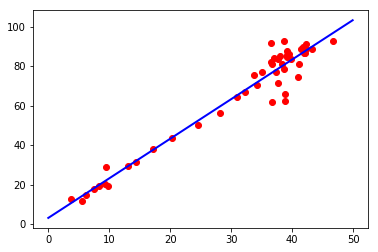I would say that our learning algorithm fits the sample data set quite well. Beside gradient descent method, we can also simply set the gradient equal zero and solve $\theta$ directly. Using matrices $A$ and $B$ from previous codes, we see that

$$\nabla J = A \theta - B.$$

So setting $\nabla J = 0$, one easily gets

$$\theta = A^{-1}B.$$
theta = np.dot(np.linalg.inv(A), B)
print(theta)

[[ 2.00547626]
[ 3.12820071]]


This is exactly the same as the one from gradient descent method! Next, I am going to show a case of linear regression with multiple features. The sample data set we are going to use is: Immigrant Salaries and Skills, U.S. 1909. You can find data here and description here.

immwork_url = 'http://www.stat.ufl.edu/~winner/data/immwork.dat'
response = requests.get(immwork_url)
data_pat = '(\d+\.\d+)'
data = map(float, re.findall(data_pat, response.content))
x = zip(data[1::4], data[2::4], data[3::4])
y = data[::4]
m = len(y)
print('Number of sample points: {}'.format(m))
print('The first three sample points:')
for i in range(3):
print(y[i], x[i])

Number of sample points: 35
The first three sample points:
(9.73, (54.9, 92.1, 54.6))
(13.07, (66.0, 96.8, 71.2))
(10.31, (20.3, 78.2, 8.5))

f, axs = plt.subplots(1, 3, sharey=True)
axs.plot(data[1::4], y, 'ro')
axs.set_title('English')
axs.set_ylabel('Wage')
axs.plot(data[2::4], y, 'go')
axs.set_title('Literacy')
axs.plot(data[3::4], y, 'bo')
axs.set_title('Tenure')
plt.show()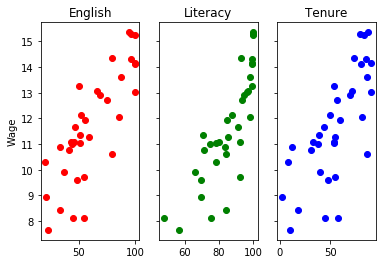Roughly, weekly wage $y$ depends linearly on each varible: English speaking ($x_1$), Literacy rate ($x_2$) and Tenure ($x_3$). For convenience, we use a dummy feature $x_0 = 1$. In general, assume that $y$ depends on $n$ features $x_1, \dots, x_n$ with one extra dummy feature $x_0 = 1$. Then

$$y = \theta^T x,$$

with

$$\theta = \left[\begin{array}{c}\theta_0 \\ \theta_1 \\ \vdots \\ \theta_n\end{array}\right], x = \left[\begin{array}{c}x_0 \\ x_1 \\ \vdots \\ x_n\end{array}\right]$$

Recall that we need to minimize

$$\begin{split} J(\theta) &= \frac{1}{2}\sum_{i=1}^{m}\left(y^{(i)}-h_\theta(x^{(i)})\right)^2 \\ &= \frac{1}{2}\sum_{i=1}^{m}\left(y^{(i)}-\sum_{j=0}^n \theta_j x_j^{(i)}\right)^2. \end{split}$$

Similar to the previous simple case, we can write $\nabla J$ in terms of matrices.

$$\nabla J = A\theta - B,$$

where

$$A_{jk} = \sum_{i=1}^m x_j^{(i)}x_k^{(i)}, B_j = \sum_{i=1}^m y^{(i)}x_j^{(i)}.$$

By setting $\nabla J = 0$,

$$\theta = A^{-1}B.$$
# number of features
n = 3
# add dummy feature to x
for i in range(m):
x[i] = (1, ) + x[i]
# take out 5 random sample points for later test
data = zip(y, x)
np.random.shuffle(data)
test = data[:5]
x = map(lambda t: t, data[5:])
y = map(lambda t: t, data[5:])

# update the size of the training set
m = len(x)

# set up matrices A and B
A = np.empty((n+1, n+1), dtype=np.float64)
for j in range(n+1):
for k in range(n+1):
A[j][k] = sum(x[i][j]*x[i][k] for i in range(m))
B = np.empty((n+1, 1), dtype=np.float64)
for j in range(n+1):
B[j] = sum(y[i]*x[i][j] for i in range(m))

# theta = A^{-1} * B
theta = np.dot(np.linalg.inv(A), B)
print(theta)

[[ 2.43496481]
[ 0.04632102]
[ 0.0794542 ]
[-0.00705355]]


To see how well the model fits the data, I use 5 random sample points to test the model. Each sample point has its own color. 'o' points are the observed values, while 'x' points are the predicted values. Also, we can calculate the percentage error of the prediction by the model.

# observed points
test_x = map(lambda t: t, test)
test_y = map(lambda t: t, test)
# prediction
predict_y = map(lambda t: np.dot(t, theta), test_x)

# plot these 5 points according to English speaking
c = ['r', 'g', 'b', 'y', 'k']
for j in range(5):
plt.plot([test_x[j]], [test_y[j]], c[j]+'o')
plt.plot([test_x[j]], [predict_y[j]], c[j]+'x')
plt.title('English')
plt.ylabel('Wage')
plt.show()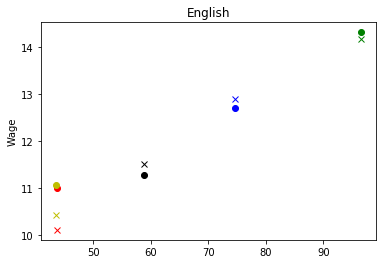# absolute error
abs_error = sum(abs(s-t) for s, t in zip(test_y, predict_y))
# percentage error
per_error = abs_error / sum(test_y)
print('The percentage error is {0:.2f}%'.format(per_error * 100))

The percentage error is 3.47%


## Logistic Regression

We are going to predict the probability of good field goals based on the dataset NFL Field Goal Attempts 2003. Let's get the data and do some plotting first.

fieldgoal_url = 'http://www.stat.ufl.edu/~winner/data/fieldgoal.dat'
response = requests.get(fieldgoal_url)
data_pat = '(\d+)'
data = map(int, re.findall(data_pat, response.content))
x, y = data[::3], data[1::3]
m = len(x)

good_x = [x[i] for i in range(m) if y[i]==1]
bad_x = [x[i] for i in range(m) if y[i]==0]
plt.hist(good_x, 30, normed=1, facecolor='green', alpha=0.75)
plt.show()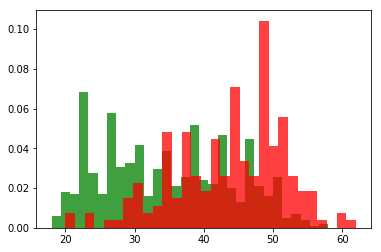There is only one feature yardage, which we will denote $x_1$. As always, we introduce a dummy feature $x_0=1$, then

$$h_\theta(x) = \frac{1}{1+e^{-\theta^Tx}},$$

where

$$x = \left[\begin{array}{c}x_0 \\ x_1\end{array}\right], \theta = \left[\begin{array}{c}\theta_0 \\ \theta_1\end{array}\right].$$

To determine $\theta$, we need to maximize the log-likelihood function

$$l(\theta) = \sum_{i=1}^m y^{(i)} \log h_\theta(x^{(i)}) + (1-y^{(i)}) \log(1-h_\theta(x^{(i)})).$$

For $\theta_j$, one can calculate $\frac{\partial l}{\partial \theta_j}$, which is

$$\frac{\partial l}{\partial \theta_j} = \sum_{i = 1}^m \left(y^{(i)} - h_\theta(x^{(i)})\right) \cdot x^{(i)}_j.$$

Note that, the gradient of $l$ is exactly in the same form as the one of $J$ in linear regression.

For the convenience of implementation for this example, let me write

$$h_{a, b}(x) = \frac{1}{1+e^{-(ax+b)}},$$

where $x$ is just $x_1$, and $a$ and $b$ together play the role of $\theta$ in the above analysis. The gradient of $l$ is now

$$\begin{split} \frac{\partial l}{\partial a} &= \sum_{i = 1}^m \left(y^{(i)} - h_{a, b}(x^{(i)})\right) \cdot x^{(i)} \\ \\ \frac{\partial l}{\partial b} &= \sum_{i = 1}^m \left(y^{(i)} - h_{a, b}(x^{(i)})\right) \end{split}$$

We observe that the range of $x^{(i)}$ is $[0, 100]$, because $x^{(i)}$ represents the yardage in a football field. Indeed, we can use min(x) and max(x) to find a more precise range of $x^{(i)}$. This observation can accerlate our calculation. Suppose gxc is a counter for all yardage of good field goals, and bxc is a counter for the bad ones. Then the gradient of $l$ can be simplified to

$$\begin{split} \frac{\partial l}{\partial a} &= \sum_{i = \min(x)}^{\max(x)} \left(\mathrm{gxc[i]} \cdot (1 - h_{a, b}(i)) + \mathrm{bxc[i]} \cdot(0 - h_{a, b}(i))\right) \cdot i \\ \\ \frac{\partial l}{\partial b} &= \sum_{i = \min(x)}^{\max(x)} \mathrm{gxc[i]} \cdot (1 - h_{a, b}(i)) + \mathrm{bxc[i]} \cdot(0 - h_{a, b}(i)) \\ \end{split}$$
import collections
gxc = collections.Counter(good_x)
min_x = min(x)
max_x = max(x)

def h(a, b, t):
eta = a*t + b
return 1.0/(1 + np.exp(-eta))

da = db = 0
for i in range(min_x, max_x+1):
ph = h(a, b, i)
t = gxc[i] * (1 - ph) + bxc[i] * (-ph)
da += t*i
db += t
return da, db

a = b = 0
alpha = 10 ** (-5)
iteration_count = 10 ** 5
for _ in range(iteration_count):
a, b = a + alpha*da, b + alpha*db
print(a, b)

(-0.14675490410919528, 6.2622768245732017)


Let's try to visualize how good our model fits the data. To this end, we recall that logistic regression gives prediction of probability of success. So we need to calculate the observed probability first, and then compare it to the model.

# calculate the observed probability
observation = []
for i in range(min_x, max_x+1):
total = gxc[i] + bxc[i]
total = 1.0 if total == 0 else float(total)
observation.append(gxc[i]/total)

# plot observation
plt.plot(range(min_x, max_x+1), observation, 'ro')

# plot prediction
u = np.arange(min_x, max_x+1, 0.1)
v = map(lambda t: h(a, b, t), u)
plt.plot(u, v, 'b', linewidth=2)

plt.show()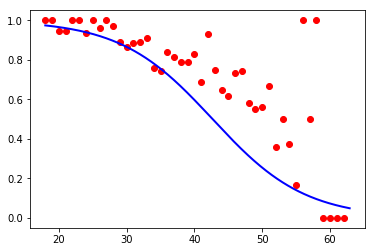It looks like that the model dose not fit well. We can simply do gradient descent again, but this time, we start from the previous calculated a and b. Since we are close to the optimal solution, we decrease the learning rate alpha to $10^{-6}$ and also decrease the iteration steps.

alpha = 10 ** (-6)
c = 10 ** 4
for _ in range(c):
a, b = a + alpha*da, b + alpha*db
print(a, b)

(-0.12228007960331008, 6.237420310313901)

# plot observation
plt.plot(range(min_x, max_x+1), observation, 'ro')

# plot prediction
u = np.arange(min_x, max_x+1, 0.1)
v = map(lambda t: h(a, b, t), u)
plt.plot(u, v, 'b', linewidth=2)

plt.show()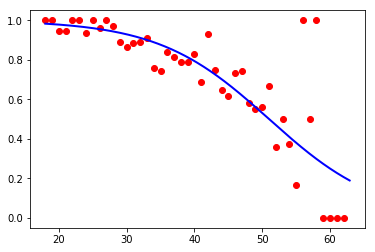Compare to the intial result, we get a better fit! Something I want to mention: when I tried to determine a and b for the first time, I forgot that I need to maximize the log-likelihood function. I used the same codes as the one for $J$ in the linear regression, which certainly did not give any good result. It takes me a long time to figure out that I actually need to use gradient ascent instead of gradient descent. These two methods differ only by a sign! Assume the learning rate $\alpha$ is always positive.

In linear regression, we use

$$\theta^{(n+1)} = \theta^{(n)} - \alpha \cdot \nabla J.$$

But in logistic regression, we should use

$$\theta^{(n+1)} = \theta^{(n)} + \alpha \cdot \nabla l.$$